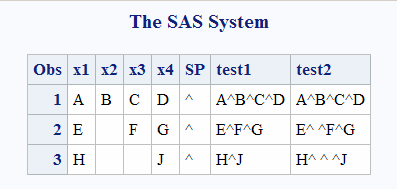# CATX Function

Removes leading and trailing blanks, inserts delimiters, and returns a concatenated character string.

 Category: Character Restriction: I18N Level 0 functions are designed for use with Single Byte Character Sets (SBCS) only.

## Syntax

CATX(delimiter, item-1 <, … item-n> )

### Required Arguments

#### delimiter

specifies a character string that is used as a delimiter between concatenated items.

#### item

specifies a constant, variable, or expression, either character or numeric. If item is numeric, then its value is converted to a character string by using the BESTw. format. In this case, SAS does not write a note to the log. For more information, see The Basics.

## Details

### The Basics

The CATX function first copies item-1 to the result, omitting leading and trailing blanks. Then for each subsequent argument item-i, i=2, …, n, if item-i contains at least one non-blank character, then CATX appends delimiter and item-i to the result, omitting leading and trailing blanks from item-i. CATX does not insert the delimiter at the beginning or end of the result. Blank items do not produce delimiters at the beginning or end of the result, nor do blank items produce multiple consecutive delimiters.

### Length of Returned Variable

In a DATA step, if the CATX function returns a value to a variable that has not previously been assigned a length, then that variable is given a length of 200 bytes. If the concatenation operator (||) returns a value to a variable that has not previously been assigned a length, then that variable is given a length that is the sum of the lengths of the values which are being concatenated.

### Length of Returned Variable: Special Cases

The CATX function returns a value to a variable, or returns a value in a temporary buffer. The value that is returned from the CATX function has the following length:
• up to 200 characters in WHERE clauses and in PROC SQL
• up to 32767 characters in the DATA step except in WHERE clauses
• up to 65534 characters when CATX is called from the macro processor
If CATX returns a value in a temporary buffer, the length of the buffer depends on the calling environment, and the value in the buffer can be truncated after CATX finishes processing. In this case, SAS does not write a message about the truncation to the log.
If the length of the variable or the buffer is not large enough to contain the result of the concatenation, SAS does the following:
• changes the result to a blank value in the DATA step, and in PROC SQL
• writes a warning message to the log stating that the result was either truncated or set to a blank value, depending on the calling environment
• writes a note to the log that shows the location of the function call and lists the argument that caused the truncation
• sets _ERROR_ to 1 in the DATA step

## Comparisons

The results of the CAT, CATS, CATT, and CATX functions are usually equivalent to results that are produced by certain combinations of the concatenation operator (||) and the TRIM and LEFT functions. However, the default length for the CAT, CATS, CATT, and CATX functions is different from the length that is obtained when you use the concatenation operator. For more information, see Length of Returned Variable.
Using the CAT, CATS, CATT, and CATX functions is faster than using TRIM and LEFT, and you can use them with the OF syntax for variable lists in calling environments that support variable lists.
Note: In the case of variables that have missing values, the concatenation produces different results. SeeConcatenating Strings That Have Missing Values.
The following table shows equivalents of the CAT, CATS, CATT, and CATX functions. The variables X1 through X4 specify character variables, and SP specifies a delimiter, such as a blank or comma.
Function
Equivalent Code
`CAT(OF X1-X4)`
`X1||X2||X3||X4`
`CATS(OF X1-X4)`
```TRIM(LEFT(X1))||TRIM(LEFT(X2))||TRIM(LEFT(X3))||
TRIM(LEFT(X4))```
`CATT(OF X1-X4)`
`TRIM(X1)||TRIM(X2)||TRIM(X3)||TRIM(X4)`
`CATX(SP, OF X1-X4)`
```TRIM(LEFT(X1))||SP||TRIM(LEFT(X2))||SP||
TRIM(LEFT(X3))||SP||TRIM(LEFT(X4))```

## Examples

### Example 1: Concatenating Strings That Have No Missing Values

The following example shows how the CATX function concatenates strings the have no missing values.
```data _null_;
separator='%%\$%%';
x='The Olympic  ';
y='   Arts Festival ';
z='   includes works by ';
a='Dale Chihuly.';
result=catx(separator,x,y,z,a);
put result \$char.;
run;```
The following line is written to the SAS log:
```----+----1----+----2----+----3----+----4----+----5----+----6----+----7
The Olympic%%\$%%Arts Festival%%\$%%includes works by%%\$%%Dale Chihuly.
```

### Example 2: Concatenating Strings That Have Missing Values

The following example shows how the CATX function concatenates strings that contain missing values.
```data one;
length x1-x4 \$1;
input x1-x4;
datalines;
A B C D
E . F G
H . . J
;
run;
data two;
set one;
SP='^';
test1=catx(sp, of x1-x4);
test2=trim(left(x1)) || sp || trim(left(x2)) || sp || trim(left(x3)) || sp ||
trim(left(x4));
run;

proc print data=two;
run;```
Using CATX with Missing Values